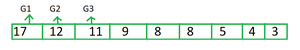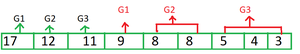GeeksforGeeks App
Open AppBrowser
Continue

# Maximize sum of minimum and maximum of all groups in distribution

Given an array arr[], and an integer N. The task is to maximize the sum of minimum and maximum of each group in a distribution of the array elements in N groups where the size of each group is given in an array b[] of size N.

Examples:

Input: a[] = {17, 12, 11, 9, 8, 8, 5, 4, 3}, N = 3,
b[] = {2, 3, 4}
Output: 60
Explanation: The array elements should be distributed in groups {17, 9} {12, 8, 8} {11, 5, 4, 3}.
So the sum becomes (17 + 9) + (12 + 8) + (11 + 3) = 26 + 20 + 14 = 60

Input: a[] = {12, 3, 4, 2, 5, 9, 8, 1, 2}, N = 3,
b[] = {1, 4, 4}
Output: 45

Approach: This problem can be solved by using the Greedy Approach and some implementation. Follow the steps below to solve the given problem.

• sort array arr[] in descending order and b[] in ascending order.
• Initialize a variable ans to store the output.
• Iterate from i = 0 to i = N-1 in array a[] and add each element to ans.
• Initialize a variable ind to store the index of elements of the array arr[]. Assign N to variable ind.
• Take a loop from i=0 to N-1.
• If b[i] > 0 then increment ind with (b[i]-1)
• Add arr[ind] to ans, as arr[ind] will be the smallest element of that group
• increment ind with 1.
• output the ans.

See the illustration below for better understanding.

Illustration:

Consider an example: arr[] = {17, 12, 11, 9, 8, 8, 5, 4, 3}, N = 3 and b[] = {2, 3, 4}

Firstly, sort array arr[] in descending order and b[] in ascending order, After then put the first N greatest element of array arr[] to each group as shown in fig.Secondly, Fill each group with the rest of the element of array arr[] (one group at a time)Therefore answer will contain the sum of the first N elements of array arr[]  i.e. 17, 12, 11 and also the last element which is filled in each group i.e. 9, 8 and 3.

Below is the implementation of the above approach.

## C++

 `// C++ program for above approach``#include ``using` `namespace` `std;` `// Function to maximum possible sum of minimum``// and maximum elements of each group``int` `geeksforgeeks(``int` `a[], ``int` `b[], ``int` `n, ``int` `k)``{``    ``// Sorting array a in descending order``    ``// and array a in ascending order.``    ``sort(a, a + n, greater<``int``>());` `    ``sort(b, b + k);` `    ``// Variable to store the required output.``    ``int` `ans = 0;` `    ``// Loop to store sum of first k greatest``    ``// element of array a[] in ans.``    ``// since they will be gretest element``    ``// of each group when distributed``    ``// in group one by one.``    ``for` `(``int` `i = 0; i < k; i++) {``        ``if` `(b[i] == 1) {``            ``ans += 2 * a[i];``        ``}``        ``else` `{``            ``ans += a[i];``        ``}` `        ``--b[i];``    ``}``    ``// Variable to store index of element a .``    ``int` `ind = k;` `    ``// Then after when each grouped is filled,``    ``// then add a[ind] to ans as a[ind] will be``    ``// lowest element of each group.``    ``for` `(``int` `i = 0; i < k; i++) {``        ``if` `(b[i] > 0) {``            ``ind += b[i] - 1;``            ``ans += a[ind];``            ``ind++;``        ``}``    ``}` `    ``return` `ans;``}` `// Driver code``int` `main()``{` `    ``int` `N = 3;` `    ``// Size of array a[]``    ``int` `siz = 9;` `    ``int` `a[] = { 17, 12, 11, 9, 8, 8, 5, 4, 3 };``    ``int` `b[] = { 2, 3, 4 };` `    ``// Function Call``    ``cout << geeksforgeeks(a, b, 9, N);``}`

## Java

 `// Java code to find the maximum median``// of a sub array having length at least K.``import` `java.util.*;``public` `class` `GFG``{` `// Utility function to sort an array in``// descending order``static` `void` `sort(``int` `arr[])``{``    ``for` `(``int` `i = ``0``; i < arr.length; i++)``    ``{    ``        ``for` `(``int` `j = i + ``1``; j < arr.length; j++)``        ``{    ``              ``if``(arr[i] < arr[j])``              ``{   ``                  ``int` `temp = arr[i];   ``                  ``arr[i] = arr[j];   ``                  ``arr[j] = temp;   ``              ``}    ``        ``}    ``    ``}   ``}` `// Function to maximum possible sum of minimum``// and maximum elements of each group``static` `int` `geeksforgeeks(``int` `a[], ``int` `b[], ``int` `n, ``int` `k)``{``    ``// Sorting array a in descending order``    ``// and array b in ascending order.``    ``sort(a);` `    ``Arrays.sort(b);` `    ``// Variable to store the required output.``    ``int` `ans = ``0``;` `    ``// Loop to store sum of first k greatest``    ``// element of array a[] in ans.``    ``// since they will be gretest element``    ``// of each group when distributed``    ``// in group one by one.``    ``for` `(``int` `i = ``0``; i < k; i++) {``        ``if` `(b[i] == ``1``) {``            ``ans += ``2` `* a[i];``        ``}``        ``else` `{``            ``ans += a[i];``        ``}` `        ``--b[i];``    ``}``    ``// Variable to store index of element a .``    ``int` `ind = k;` `    ``// Then after when each grouped is filled,``    ``// then add a[ind] to ans as a[ind] will be``    ``// lowest element of each group.``    ``for` `(``int` `i = ``0``; i < k; i++) {``        ``if` `(b[i] > ``0``) {``            ``ind += b[i] - ``1``;``            ``ans += a[ind];``            ``ind++;``        ``}``    ``}` `    ``return` `ans;``}` `// Driver code``public` `static` `void` `main(String args[])``{``    ``int` `N = ``3``;` `    ``// Size of array a[]``    ``int` `siz = ``9``;` `    ``int` `a[] = { ``17``, ``12``, ``11``, ``9``, ``8``, ``8``, ``5``, ``4``, ``3` `};``    ``int` `b[] = { ``2``, ``3``, ``4` `};` `    ``// Function Call``    ``System.out.println(geeksforgeeks(a, b, ``9``, N));``}``}` `// This code is contributed by Samim Hossain Mondal.`

## Python

 `# Python program for the above approach` `# Function to maximum possible sum of minimum``# and maximum elements of each group``def` `geeksforgeeks(a, b, n, k):``    ` `    ``# Sorting array a in descending order``    ``# and array a in ascending order.``    ``a.sort(reverse``=``True``)` `    ``b.sort()` `    ``# Variable to store the required output.``    ``ans ``=` `0` `    ``# Loop to store sum of first k greatest``    ``# element of array a[] in ans.``    ``# since they will be gretest element``    ``# of each group when distributed``    ``# in group one by one.``    ``for` `i ``in` `range``(``0``, k):``        ``if` `(b[i] ``=``=` `1``):``            ``ans ``=` `ans ``+` `(``2` `*` `a[i])``        ` `        ``else``:``            ``ans ``=` `ans ``+` `a[i]` `        ``b[i] ``=` `b[i] ``-` `1``        ` `    ``# Variable to store index of element a .``    ``ind ``=` `k` `    ``# Then after when each grouped is filled,``    ``# then add a[ind] to ans as a[ind] will be``    ``# lowest element of each group.``    ``for` `i ``in` `range``(``0``, k):``        ``if` `(b[i] > ``0``):``            ``ind ``=` `ind ``+` `b[i] ``-` `1``            ``ans ``=` `ans ``+` `a[ind]``            ``ind ``=` `ind ``+` `1` `    ``return` `ans` `# Driver code``N ``=` `3` `# Size of array a[]``siz ``=` `9``;` `a ``=` `[ ``17``, ``12``, ``11``, ``9``, ``8``, ``8``, ``5``, ``4``, ``3` `]``b ``=` `[ ``2``, ``3``, ``4` `]` `# Function Call``print``(geeksforgeeks(a, b, ``9``, N))` `# This code is contributed by Samim Hossain Mondal.`

## C#

 `// C# code to find the maximum median``// of a sub array having length at least K.``using` `System;``public` `class` `GFG``{` `  ``// Utility function to sort an array in``  ``// descending order``  ``static` `void` `sort(``int``[] arr)``  ``{``    ``for` `(``int` `i = 0; i < arr.Length; i++)``    ``{    ``      ``for` `(``int` `j = i + 1; j < arr.Length; j++)``      ``{    ``        ``if``(arr[i] < arr[j])``        ``{   ``          ``int` `temp = arr[i];   ``          ``arr[i] = arr[j];   ``          ``arr[j] = temp;   ``        ``}    ``      ``}    ``    ``}   ``  ``}` `  ``// Function to maximum possible sum of minimum``  ``// and maximum elements of each group``  ``static` `int` `geeksforgeeks(``int``[] a, ``int``[] b, ``int` `n, ``int` `k)``  ``{``    ` `    ``// Sorting array a in descending order``    ``// and array b in ascending order.``    ``sort(a);` `    ``Array.Sort(b);` `    ``// Variable to store the required output.``    ``int` `ans = 0;` `    ``// Loop to store sum of first k greatest``    ``// element of array a[] in ans.``    ``// since they will be gretest element``    ``// of each group when distributed``    ``// in group one by one.``    ``for` `(``int` `i = 0; i < k; i++) {``      ``if` `(b[i] == 1) {``        ``ans += 2 * a[i];``      ``}``      ``else` `{``        ``ans += a[i];``      ``}` `      ``--b[i];``    ``}``    ``// Variable to store index of element a .``    ``int` `ind = k;` `    ``// Then after when each grouped is filled,``    ``// then add a[ind] to ans as a[ind] will be``    ``// lowest element of each group.``    ``for` `(``int` `i = 0; i < k; i++) {``      ``if` `(b[i] > 0) {``        ``ind += b[i] - 1;``        ``ans += a[ind];``        ``ind++;``      ``}``    ``}` `    ``return` `ans;``  ``}` `  ``// Driver code``  ``public` `static` `void` `Main()``  ``{``    ``int` `N = 3;` `    ``// Size of array a[]``    ``int` `siz = 9;` `    ``int``[] a = { 17, 12, 11, 9, 8, 8, 5, 4, 3 };``    ``int``[] b = { 2, 3, 4 };` `    ``// Function Call``    ``Console.Write(geeksforgeeks(a, b, 9, N));``  ``}``}` `// This code is contributed by Saurabh Jaiswal`

## Javascript

 ``

Output

`60`

Time complexity: O(M * logM) where M is the size of array arr.
Auxiliary Space: O(1)

My Personal Notes arrow_drop_up